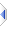# sign

 Definition: integer i = sign(atom a) Description: returns -1, 0, or +1 if a is negative, zero, or positive respectively. Comments: sign(a) returns the same result as compare(a,0). This function may be applied to an atom or sq_sign() to all elements of a sequence. The rules for sequence operations apply. Example: ```r = sign(5) -- r is 1 r = sign(0) -- r is 0 r = sign(-2.5) -- r is -1 ``` Implementation: See builtins\pmaths.e (an autoinclude) for details of the actual implementation. See Also: compare